New Students Offer - Use Code HELLO

# Average Formulas & Shortcuts For Bank Exams1. Common Formula used to calculate average

Ex. What is the average of 10, 11, 13, 15, 18, and 23?
Solution:
Sum of numbers= 10+11+13+15+18+23 = 90
Total numbers = 6
Average = 90/6 =15

2. If the difference between the numbers is consonant or same, like 2,4,6,8 or 3, 6, 9, 12 then

Ex. Find the average of 4, 8, 12, 16, 20, and 24.
Solution:
The difference between the numbers is consonant or same.
=14

3. Average of the first ‘n’ consecutive natural numbers is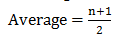Ex. What is the average of the first 25consecutive natural numbers?
Solution:
=13

2nd Method:
1,2,3,4,5,6,7,8,9,.....,20,21,22,23,24,25
Sum of numbers = 325, Total numbers = 25=13

4. Average of the first ‘n’ natural even number is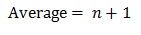Ex. What is the average of the first 10 natural even numbers?
Solution:
Average= 10+1 =11

2nd Method:
2,4,6,8,10,12,14,16,18,20
Sum of numbers= 110, Total numbers= 10
=11

5. Average of the first ‘n’natural odd numbers is
Average = n

Ex. What is the average of the first 8 natural odd numbers?
Solution:
Average = 8
2nd Method:
1,3,5,7,9,11,13,15
Sum of numbers = 64, Total numbers = 8

=8

6. Average of natural even numbers up to ‘n’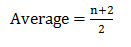Ex. What is the average of the even numbers up to 22?
Solution:Average = 12

2nd Method:
2,4,6,8,10,12,14,16,18,20,22
Sum of numbers = 132, Total numbers= 11

=12

7. Average of natural odd numbers up to ‘n’

Ex. What is the average of the odd numbers up to 17?
Solution:
Average = 9

2nd Method:
1,3,5,7,9,11,13,15,17
Sum of numbers = 81, Total numbers= 9
=9
8. Average of squares of first ‘n’ natural numbers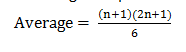Ex. What is the average of the squares of the first 10 natural numbers?
Solution:Average= 38.5

2nd Method:

1,4,9,16,25,36,49,64,81,100
Sum of numbers=385, Total number=10
Average= 38.5

9. Average of the cubes of first ‘n’ natural numbersEx. What is the average of the cubes of the first 6 natural numbers?
Solution:

Average= 73.5

2nd Method:
1,8,27,64,125,216
Sum of numbers=385, Total number=6
Average= 73.5

10. Average of ‘n’ multiple of ‘a’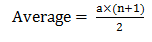Ex. What is the average of 6 multiple of 5?
Solution:

Average= 17.5

2nd Method:
5,10,15,20,25,30
Sum of numbers=105, Total numbers=6Average= 17.5